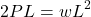# Online Embedded Excel Aug 2023

My first online embedded Excel spreadsheet
Grating Calculation
I present a grating calculation spreadsheet as an example of an embedded Excel application

Input cells (red font) may be freely edited by the user
Calculated cells provide numeric results (cell formulas are hidden)
Compare my calculations to the load table PDF from Borden Metal Products (Canada) Ltd.

• The spreadsheet basis is a one-foot width of grating
• Grating is welded, not "press-locked"
• Grating is "built" from user dimensional input (∴ not proprietary)
• The clear span is calculated from 2 user inputs, center-to-center span and support width
• The maximum allowable deflection is set at 0.25 inch (petrochemical industry standard)
• The effect of grating weight is considered in calculating allowable loads:
• the uniform weight of grating is deducted from the maximum uniform load
• the "equivalent concentrated load" is deducted from the maximum concentrated load
(moment basis, for example)

From Mechanics of Solids:Set:Therefore,And finally,Therefore, if the weight of grating is say 16 pounds/foot (0.016 kips/foot),
the basis is a one-foot width of grating,
and the clear span is say 5′, thenAnd(deflection basis, for example)

From Mechanics of Solids:Set:Therefore,And finally,Therefore, if the weight of grating is say 16 pounds/foot (0.016 kips/foot),
the basis is a one-foot width of grating,
and the clear span is say 5′, thenAnd